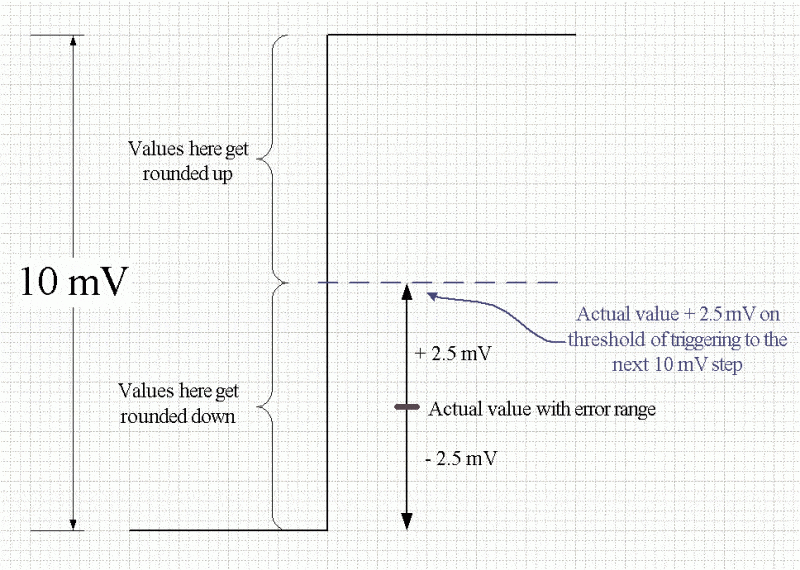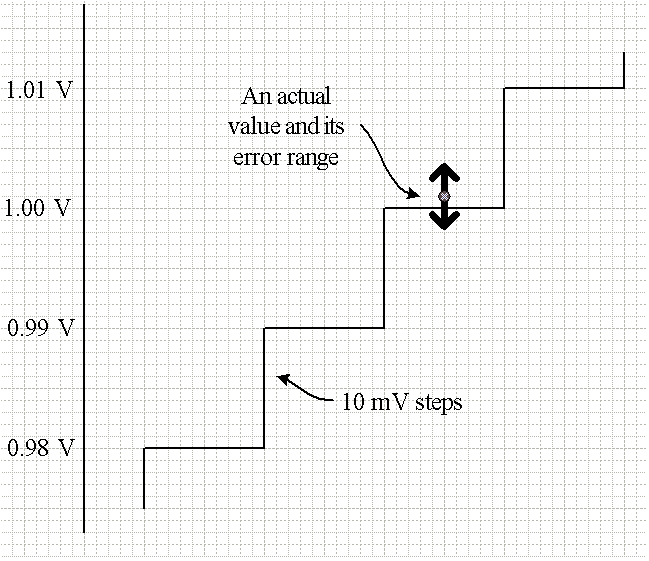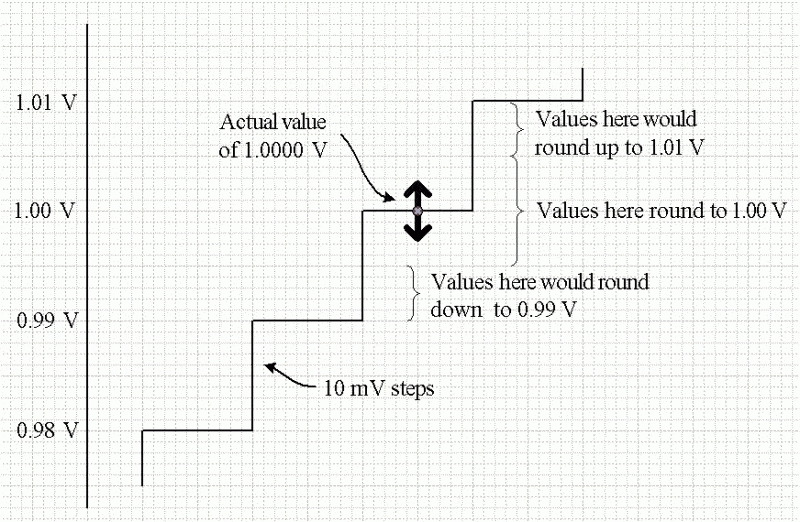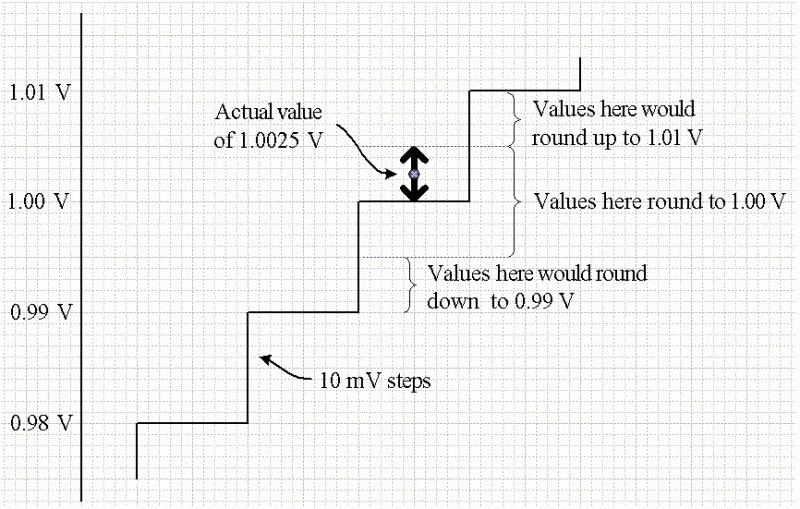# Data problem for acquisition

• JI567

## Homework Statement

Question is determine the maximum amount by which the Analogue to digital converter output can differ from the analogue input when a 8-bit Analogue to digital converter has a full scale input of 2.55 V. It has a specified error of 0.1% full - Scale.

## Homework Equations

% resolution = step size/ full scale *100%

## The Attempt at a Solution

I just did 0.1% * 2.55 to get the specified error which was 2.5*10^-3. Then I calculated the resolution which was (2.55/2^8-1)*100% = 0.01. So for each resolution the error was 2.5*10^-3. Thus, maximum amount by which it could differ was 0.01*2.5*10^-3 = 2.5*10^-5. Is this correct at all? Please help...!

## Homework Statement

Question is determine the maximum amount by which the Analogue to digital converter output can differ from the analogue input when a 8-bit Analogue to digital converter has a full scale input of 2.55 V. It has a specified error of 0.1% full - Scale.

## Homework Equations

% resolution = step size/ full scale *100%

## The Attempt at a Solution

I just did 0.1% * 2.55 to get the specified error which was 2.5*10^-3. Then I calculated the resolution which was (2.55/2^8-1)*100% = 0.01. So for each resolution the error was 2.5*10^-3. Thus, maximum amount by which it could differ was 0.01*2.5*10^-3 = 2.5*10^-5. Is this correct at all? Please help...!

You correctly calculated 0.1% of full scale as 2.55mV. And the resolution is 10mV per step as well. Calculating the overall accuracy is a bit trickier, depending on how you want to define it.

As long as the output of the ADC is monotonic (no missing codes, etc.), then the overall error would be how early/late the output code is versus a swept input voltage. Does your textbook show you a figure of how the overall error is calculated versus the step size of the ADC?

You correctly calculated 0.1% of full scale as 2.55mV. And the resolution is 10mV per step as well. Calculating the overall accuracy is a bit trickier, depending on how you want to define it.

As long as the output of the ADC is monotonic (no missing codes, etc.), then the overall error would be how early/late the output code is versus a swept input voltage. Does your textbook show you a figure of how the overall error is calculated versus the step size of the ADC?

Nope no diagrams on textbook. This question is all I was given. How do I calculate the maximum amount? Do I just add up the 2.55 mV and 10 mV?

Nope no diagrams on textbook. This question is all I was given. How do I calculate the maximum amount? Do I just add up the 2.55 mV and 10 mV?

Have a look at this tutorial from Maxim. Especially check out the ADC Gain Error and INL definitions to see if they help...

http://www.maximintegrated.com/en/app-notes/index.mvp/id/641

:-)

They don't unfortunately...Are you sure its really so complicated? We didn't study those things in the lectures...aren't there some easy formulas to solve this question?

Hi JI567,

While it's not clear to me what the specifications of accuracy and resolution are meant to imply in the context of your course, I was thinking that it might be worthwhile considering what happens when an actual value lands somewhere in a 10 mV step range of the converter but has an error of +/- 2.5 mV associated with it. How far away from the the true value might the ADC's reading be if it has to round up or down to a step? A sketch might help.

Hi JI567,

While it's not clear to me what the specifications of accuracy and resolution are meant to imply in the context of your course, I was thinking that it might be worthwhile considering what happens when an actual value lands somewhere in a 10 mV step range of the converter but has an error of +/- 2.5 mV associated with it. How far away from the the true value might the ADC's reading be if it has to round up or down to a step? A sketch might help.

if you are talking about the +/- range then shouldn't it just be 2*2.5 mV?

if you are talking about the +/- range then shouldn't it just be 2*2.5 mV?
What's your reasoning? As I mentioned, a sketch of the situation may be helpful. Look for the worst case situation.

What's your reasoning? As I mentioned, a sketch of the situation may be helpful. Look for the worst case situation.

a sketch of what though? Like what on the y-axis and what on the x axis?

Sketch one step of the ADC's "stairstep" transfer function (a 10 mV jump between output values). Imagine that a measured value falls somewhere in that range so that the when the ADC does the conversion it must round either up or down to the nearest 10 mV level. But the input value has an error of +/- 2.5 mV associated with it, so for a given actual value it could fall anywhere within a spread of values on the sketch. Find the worst case scenario for the difference between the actual value and the "measured" value.

Sketch one step of the ADC's "stairstep" transfer function (a 10 mV jump between output values). Imagine that a measured value falls somewhere in that range so that the when the ADC does the conversion it must round either up or down to the nearest 10 mV level. But the input value has an error of +/- 2.5 mV associated with it, so for a given actual value it could fall anywhere within a spread of values on the sketch. Find the worst case scenario for the difference between the actual value and the "measured" value.

A measured value falls within a range of 10 mV? I mean how am I supposed to sketch it? I don't have any other points or values. Sorry I haven't been taught stairstep transfer function in lectures. But anyways why don't we just write 10mv - 2.5 mv as the measured value for the worst case scenario then? As for the actual value I don't know what it is as its not given in the question.

A measured value falls within a range of 10 mV? I mean how am I supposed to sketch it? I don't have any other points or values.
The resolution of the ADC is 10 mV --- that's the value that you calculated. It can only return values in 10 mV increments. Any input value applied to the ADC will fall within some 10 mV step, and will be rounded to the nearest 10 mV value. So an input of 1.322 V would be rounded to the 1.23 level, while an input of 1.326 would round to the 1.33 level.
Sorry I haven't been taught stairstep transfer function in lectures.
Picture a staircase with a slope of 45 degrees. Each step has a height of 10 mV. Each level corresponds to one of the 28 possible outputs of the ADC.
But anyways why don't we just write 10mv - 2.5 mv as the measured value for the worst case scenario then? As for the actual value I don't know what it is as its not given in the question.
You don't need a particular actual value. You propose some value that will fall somewhere in the ADC's input range. A particular input value isn't important. It's the fact that any input value will be rounded to the nearest 10 mV level.

So yes, 10 mV - 2.5 mV sounds like a reasonable worst-case scenario:The resolution of the ADC is 10 mV --- that's the value that you calculated. It can only return values in 10 mV increments. Any input value applied to the ADC will fall within some 10 mV step, and will be rounded to the nearest 10 mV value. So an input of 1.322 V would be rounded to the 1.23 level, while an input of 1.326 would round to the 1.33 level.

Picture a staircase with a slope of 45 degrees. Each step has a height of 10 mV. Each level corresponds to one of the 28 possible outputs of the ADC.

You don't need a particular actual value. You propose some value that will fall somewhere in the ADC's input range. A particular input value isn't important. It's the fact that any input value will be rounded to the nearest 10 mV level.

So yes, 10 mV - 2.5 mV sounds like a reasonable worst-case scenario:

View attachment 77089

Alright that's some new information...so what's the answer to the question? 7.5 mV?

Alright that's some new information...so what's the answer to the question? 7.5 mV?
By Forum rules I can't give you an answer to that... Homework Helpers are not allowed to provide solutions directly. We can provide hints, corrections, and background information to help you arrive at a solution yourself. I can state that I don't find any fault with your reasoning :)

By Forum rules I can't give you an answer to that... Homework Helpers are not allowed to provide solutions directly. We can provide hints, corrections, and background information to help you arrive at a solution yourself. I can state that I don't find any fault with your reasoning :)

Why shouldn't the answer be 12.5 mV? I mean isn't that the maximum value obtained? I thought that's what the question was asking for.

Why shouldn't the answer be 12.5 mV? I mean isn't that the maximum value obtained? I thought that's what the question was asking for.
Can you sketch a situation where an input would lead to a 12.5 mV difference? Remember, the ADC will round to the nearest 10 mV level.

Can you sketch a situation where an input would lead to a 12.5 mV difference? Remember, the ADC will round to the nearest 10 mV level.

so you mean because the resolution of the ADC is 10 mV the difference can't be higher than 10mV but lower than it?

so you mean because the resolution of the ADC is 10 mV the difference can't be higher than 10mV but lower than it?
Yes, since the possible error in the input value is less than half a step size. There's no input value that would put its error range more than a half-step away, which is what would be required to jump a whole 10 mV away:Slide the actual value and its error range up and down on the stairstep and see what values the extremes of the error range would round to.

Yes, since the possible error in the input value is less than half a step size. There's no input value that would put its error range more than a half-step away, which is what would be required to jump a whole 10 mV away:

View attachment 77090

Slide the actual value and its error range up and down on the stairstep and see what values the extremes of the error range would round to.

well let's say at 1 V. For the upper part will it be 1.0025 V? which will be rounded to 1.003? and for the down part it will be 0.9975 so will be rounded to 0.998?

well let's say at 1 V. For the upper part will it be 1.0025 V? which will be rounded to 1.003? and for the down part it will be 0.9975 so will be rounded to 0.998?
10 mV is 0.01 V. So you get two decimal digits in any rounded result. Both of those values would round to 1.00 V, the nearest step.10 mV is 0.01 V. So you get two decimal digits in any rounded result. Both of those values would round to 1.00 V, the nearest step.

View attachment 77091

Okay so for this question each resolution of 10 mV has an maximum and minimum error of 2.5 mV. Since the error in input is not half step size so it will always be rounded back to 10 mV so we can't have 12.5 mV. But how are we going to have 7.5 mV? I mean that also gets rounded to 10 mV. so shouldn't the answer just be 10 mV?

Take another look at the image in post #12. If that actual value is nudged upwards just a tiny, tiny bit, what will be the difference between the actual value and the value reported by the ADC if the error happens to be at the top of its range?

Take another look at the image in post #12. If that actual value is nudged upwards just a tiny, tiny bit, what will be the difference between the actual value and the value reported by the ADC if the error happens to be at the top of its range?

it gets triggered to the next 10 mV step. The difference will be the actual value + 2.5 mv error - actual value. right?

it gets triggered to the next 10 mV step. The difference will be the actual value + 2.5 mv error - actual value. right?
No, it will be the step value that is reported minus the actual value (well, technically its the difference between the actual value and reported value. The same situation could be set up for the bottom of the error range of the value, resulting in a rounding down to the next lower step).

No, it will be the step value that is reported minus the actual value (well, technically its the difference between the actual value and reported value. The same situation could be set up for the bottom of the error range of the value, resulting in a rounding down to the next lower step).

So in the diagram if the actual value is 1.00 V then what's its reported step value?

So in the diagram if the actual value is 1.00 V then what's its reported step value?
What's the nearest 10 mV step to 1.00 V + 2.5 mV or 1.00 V - 2.5 mV?

What's the nearest 10 mV step to 1.00 V + 2.5 mV or 1.00 V - 2.5 mV?

1? So if 1 is the step value and 1+2.5 mV is the actual value? the difference is just 2.5 mV. Is that correct?

1? So if 1 is the step value and 1+2.5 mV is the actual value? the difference is just 2.5 mV. Is that correct?

Place your actual value on the stair diagram and check where the ends of the error range fall.Note that the top of the error range just touches the level where the ADC would choose to round upwards to 1.01 V. Since error ranges are not hard values, there is a possibility that your 1 V + 2.5 mV = 1.0025 V actual value could be reported as 1.01 V. That would make the difference between actual and reported value 7.5 mV, correct?

Place your actual value on the stair diagram and check where the ends of the error range fall.

View attachment 77092

Note that the top of the error range just touches the level where the ADC would choose to round upwards to 1.01 V. Since error ranges are not hard values, there is a possibility that your 1 V + 2.5 mV = 1.0025 V actual value could be reported as 1.01 V. That would make the difference between actual and reported value 7.5 mV, correct?

That is if you are going upwards. What if you are going downwards for the error? Then it just gets round to 1 and difference is 2.5 mV.

Correct.

Correct.

Ohhh Now i see why the maximum difference is 7.5 mV! I have been thinking you were just doing 10+2.5 and 10-2.5 like how they do for uncertainity range and you just chose the 10-2.5 because resolution was 10 mV. But well post 28 explains the real thing. Cheers!

P.S. how good are you with Dittus Boelter correlation and dimonsionless groups?

P.S. how good are you with Dittus Boelter correlation and dimonsionless groups?
Never touched them before. But it could be that other Homework Helpers here have dealt with them.